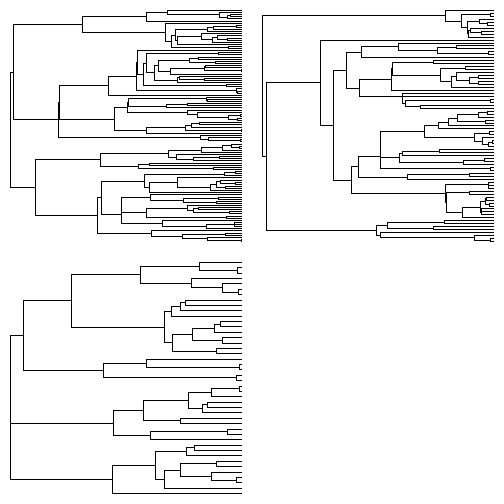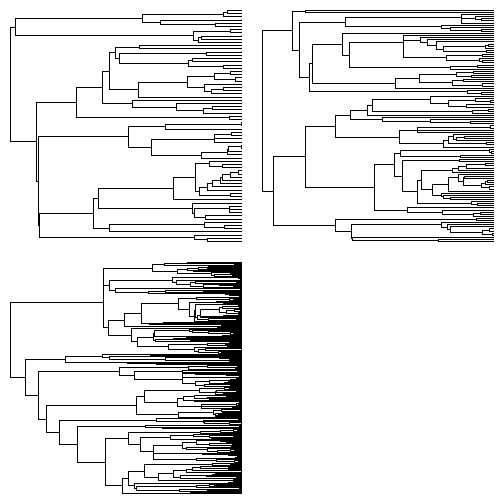## Wednesday, November 8, 2017

### Comparing the rate of diversification among trees under a 'Yule' or pure-birth model

I just pushed another update to the function `ratebytree` for comparing diversification rates among trees - in this case to permit users to fit the 'Yule' or pure-birth model. In this case, two models are fit & compared: one in which each tree is permitted to have its own speciation rate & a second where all trees are constrained to the same rate.

Here is an example with two sets of trees - one (`t1`) simulated under the null hypothesis of pure-birth & no difference in rate among trees, and the second (`t2`) simulated with a 25% higher diversification rate in tree 3 compared to trees 1 & 2:

``````library(phytools)
source("https://raw.githubusercontent.com/liamrevell/phytools/cd787d598150ac216fdbe87a60efb105e3138f0e/R/ratebytree.R")
print(t1,details=T)
``````
``````## 3 phylogenetic trees
## tree 1 : 110 tips
## tree 2 : 79 tips
## tree 3 : 44 tips
``````
``````par(mfrow=c(2,2))
plotTree(t1,ftype="off",lwd=1)
````````````ratebytree(t1,model="Yule")
``````
``````## ML common diversification-rate model:
##          b       d   k   log(L)
## value    0.037   0   1   -183.4468
##
## ML multi diversification-rate model:
##          b    b    b    d    d    d    k   log(L)
## value    0.0378  0.0396  0.0315  0   0   0   3   -182.6673
##
## Diversification model was "Yule".
## Model fitting method was "optimize".
##
## Likelihood ratio: 1.5591
## P-value (based on X^2): 0.4586
``````
``````print(t2,details=T)
``````
``````## 3 phylogenetic trees
## tree 1 : 73 tips
## tree 2 : 111 tips
## tree 3 : 235 tips
``````
``````par(mfrow=c(2,2))
plotTree(t2,ftype="off",lwd=1)
````````````ratebytree(t2,model="Yule")
``````
``````## ML common diversification-rate model:
##          b       d   k   log(L)
## value    0.0427  0   1   -19.3546
##
## ML multi diversification-rate model:
##          b    b    b    d    d    d    k   log(L)
## value    0.0355  0.0371  0.0494  0   0   0   3   -14.5664
##
## Diversification model was "Yule".
## Model fitting method was "optimize".
##
## Likelihood ratio: 9.5763
## P-value (based on X^2): 0.0083
``````

Neat. We can also compare these to alternative models, such as, for instance, our multi-rate birth-death model, using our `AIC` method. For instance:

``````yule<-ratebytree(t2,model="Yule")
bd<-ratebytree(t2,model="birth-death")
``````
``````## Warning in nlminb(c(init.b, init.d), lik.bd, t = T, rho = rho, lower =
## rep(0, : NA/NaN function evaluation

## Warning in nlminb(c(init.b, init.d), lik.bd, t = T, rho = rho, lower =
## rep(0, : NA/NaN function evaluation

## Warning in nlminb(c(init.b, init.d), lik.bd, t = T, rho = rho, lower =
## rep(0, : NA/NaN function evaluation
``````
``````AIC(yule,bd)
``````
``````##                             AIC df
## common-rate            40.70918  1
## multi-rate:Yule        35.13287  3
## multi-rate:birth-death 40.72992  6
``````

Although in this case our simplest model is just our Yule model and we have kind of ignored our birth-death null model (though we shouldn't have - I'll fix that later).

The trees were simulated using phytools as follows:

``````t1<-pbtree(b=0.039,t=100,nsim=3)
t2<-c(pbtree(b=0.039,t=100,nsim=2),
as.multiPhylo(pbtree(b=0.049,t=100)))
``````